# ISEE Middle Level Math : How to find the perimeter of a triangle

## Example Questions

### Example Question #11 : Geometry

Find the perimeter of an equilateral triangle that has a base of length 9cm.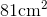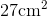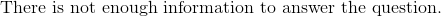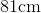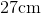Explanation:

To find the perimeter of a triangle, we will use the following formula: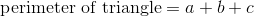where a, b, and c are the lengths of the sides of the triangle.

We know we have an equilateral triangle.  This means that all of the sides of the triangle are equal or the same length.  We know the base has a length of 9cm.  Therefore, all sides are 9cm.  Knowing this, we can substitute into the formula.  We get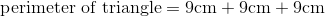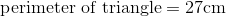### Example Question #12 : Geometry

At your local university there is a green area where students like to hang out. It is in the shape of a triangle and is bordered by walking paths. If the paths are 300ft, 400ft, and 500ft long, what is the perimeter of the triangle formed by the paths?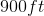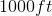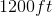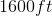Explanation:

At your local university there is a green area where students like to hang out. It is in the shape of a triangle and is bordered by walking paths. If the paths are 300ft, 400ft, and 500ft long, what is the perimeter of the triangle formed by the paths?

This problem describes a triangle and asks us to find its perimeter. To do so, we simply need to add up all of the sides.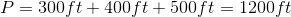So the distance around the triangle is simply 1200ft

### Example Question #13 : Geometry

The roof of a skyscraper forms a right triangle with equal arms of length 50 meters. Find the perimeter of the roof of the skyscraper.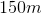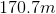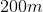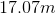Explanation:

The roof of a skyscraper forms a right triangle with equal arms of length 50 meters. Find the perimeter of the roof of the skyscraper.

To find the perimeter of a triangle, we need to know the lengths of all three sides.

We know two, and we know that it is a right triangle. If you are really savvy, you can see that this must be a 45/45/90 triangle, and therefore, the remaining side must be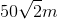You can also find this via Pythagorean Theorem: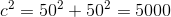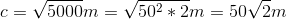Next, add it all up to get: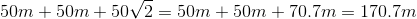Note that we rounded slightly, but our answer choices take this into account.

### Example Question #14 : Geometry

Use the following to answer the question.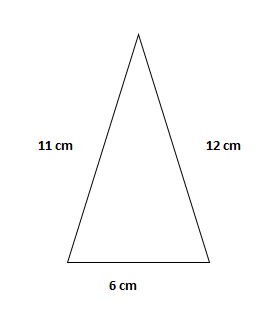Find the perimeter of the triangle.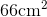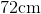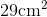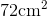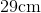Explanation:

To find the perimeter of a triangle, we will use the following formula:where a, b, and c are the lengths of the sides of the triangle.

Now, given the trianglewe can see the sides are 11cm, 12cm, and 6cm.  Knowing this, we can substitute into the formula.  We get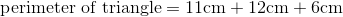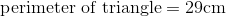### Example Question #15 : Geometry

Find the perimeter of an equilateral triangle that has a base of 3 feet.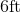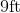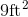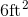Explanation:

To find the perimeter of a triangle, we will use the following formula:where a, b, and c are the lengths of the sides of the triangle.

Now, we know the base of the triangle is 3 feet.  Because it is an equilateral triangle, we know that all sides are equal.  Therefore, all sides are 3 feet.  Knowing this, we can substitute into the formula.  We get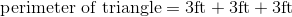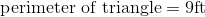### Example Question #16 : Geometry

An equilateral triangle is formed by the base of a desk. If one side of the base of the desk is 3 feet, what is the perimeter of the desk?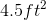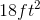Cannot be determined from the information provided.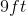Explanation:

An equilateral triangle is formed by the base of a desk. If one side of the base of the desk is 3 feet, what is the perimeter of the desk?

To find the perimeter of a shape, add up the lengths of its sides. In this case, we need to recall what equilateral means.

An equilateral triangle has three equal sides, therefore, this triangle will have 3 sides that are each 3 feet. So, we can find our answer as follows: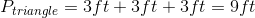### Example Question #17 : Geometry

Find the perimeter of an equilateral triangle with a base of length 7 inches.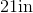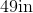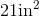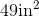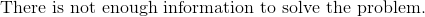Explanation:

To find the perimeter of a triangle, we will use the following formula:where a, b, and c are the lengths of the sides of the triangle.

So, we know the base of the triangle has a length of 7 inches.  Because it is an equilateral triangle, we know that all sides are equal.  Which means all sides are 7 inches.  Knowing this, we can substitute into the formula.  We get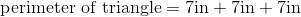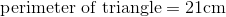### Example Question #18 : Geometry

Find the perimeter of an equilateral triangle with a base of 6.5cm.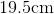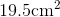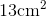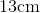Explanation:

To find the perimeter of a triangle, we will use the formula:where a, b, and c are the lengths of the sides of the triangle.

Now, we know the base of the triangle is 6.5cm.  Because it is an equilateral triangle, we know that all sides are equal.  Therefore, all sides are 6.5cm.  Knowing this, we can substitute into the formula.  We get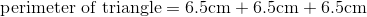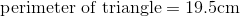### Example Question #19 : Geometry

Find the perimeter of an equilateral triangle with a base of 11cm.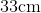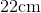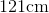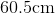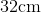Explanation:

To find the perimeter of a triangle, we will use the following formula:where a, b, and c are the lengths of the sides of the triangle.

Now, we know that the base of the triangle is 11cm.  Because it is an equilateral triangle, we know that all sides are equal.  Therefore, all sides are 11cm.

Knowing this, we can substitute into the formula.  We get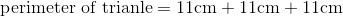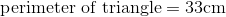### Example Question #20 : Geometry

Find the perimeter of an equilateral triangle with a base of 9cm.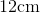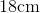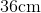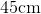Explanation:

To find the perimeter of a triangle, we will use the following formula:where a, b, and c are the lengths of the sides of the triangle.

Now, we know the base of the triangle is 9cm.  Because it is an equilateral triangle, all sides are equal.  Therefore, all sides are 9cm.

Knowing this, we can substitute into the formula.